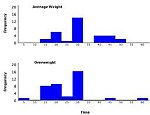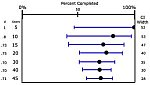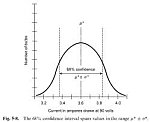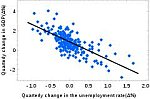# Solved Statistics Problems – Practice Problems to prepare for your exams

In this section we present a collection of solved statistics problem, with fairly complete solutions. Ideally you can use these problems to practice any statistics subject that you are in need of, for any practicing purpose, such as stats homework or tests.

___PHP___5

The collection contains solved statistic problems of various different areas in statistics, such as Descriptive Statistics, Confidence Intervals, Calculation of Normal Probabilities, Hypothesis Testing, Correlation and Regression, and Analysis of Variance (For a list of 30,00+ step-by-step solved math problems, click here )

### Descriptive Statistics### Confidence Intervals___PHP___5

### Calculation of Normal Probabilities### Hypothesis Testing### Correlation and Regression Analysis### Analysis of Variance___PHP___5